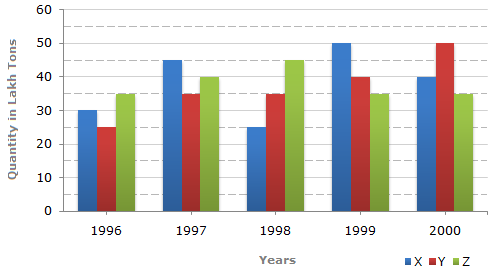# Data Interpretation - Bar Charts - Discussion

The bar graph given below shows the data of the production of paper (in lakh tonnes) by three different companies X, Y and Z over the years.

Production of Paper (in lakh tonnes) by Three Companies X, Y and Z over the Years.1.

For which of the following years, the percentage rise/fall in production from the previous year is the maximum for Company Y?

 [A]. 1997 [B]. 1998 [C]. 1999 [D]. 2000

Explanation:

Percentage change (rise/fall) in the production of Company Y in comparison to the previous year, for different years are:

 For 1997 =(35 - 25) x 100% = 40%. 25

 For 1998 =(35 - 35) x 100% = 0%. 35

 For 1999 =(40 - 35) x 100% = 14.29%. 35

 For 2000 =(50 - 40) x 100% = 25%. 40

Hence, the maximum percentage rise/fall in the production of Company Y is for 1997.

 Shruthi said: (Dec 10, 2010) I want to know how is data taken as 32 for 1997 and 35 for 1998 when it is shown clearly that for both years it is 35 please do explain.

 Shilpi said: (Jun 10, 2011) Can you please explain why is it divided by 25, 25, 35, 40?

 Mani Maran said: (Aug 24, 2011) Here. [ (current year profit) - (previous year prfit) /previous year Profit) ]*100.

 Mohammed Abbas said: (May 20, 2012) For 1998 I think it should be divided by 35. The final answer will be the same.

 Am Kumar said: (Jun 3, 2012) For product X, from 1998 to 1999 rise is from 25 to 50 ie 100%.

 Mojo said: (Oct 6, 2014) What's the difference between percentage increase/decrease and rice/fall? Formula for both is different.

 Vinod Sake said: (Jul 4, 2015) I would like to know, why are the percentage increase for year 1997 & 2001 different. By applying the formula we have got % increase. But if we see the graph, it is clearly visible that for both the years quantity has increased by 10. Anyone please explain my question? Thanks in Advance.

 Manju said: (Mar 26, 2016) Why we can't take X or Z companies.

 Mike said: (Aug 17, 2016) @Manju. Because the question was asked for Y companies.

 Bhavesh said: (Feb 12, 2018) What about year 96?

 Tshering Wangmo said: (Aug 10, 2018) For 1997, how come information of 1996 is used. Can anyone explain?

 Yeshi said: (Jul 11, 2019) What is the formula to calculate this?